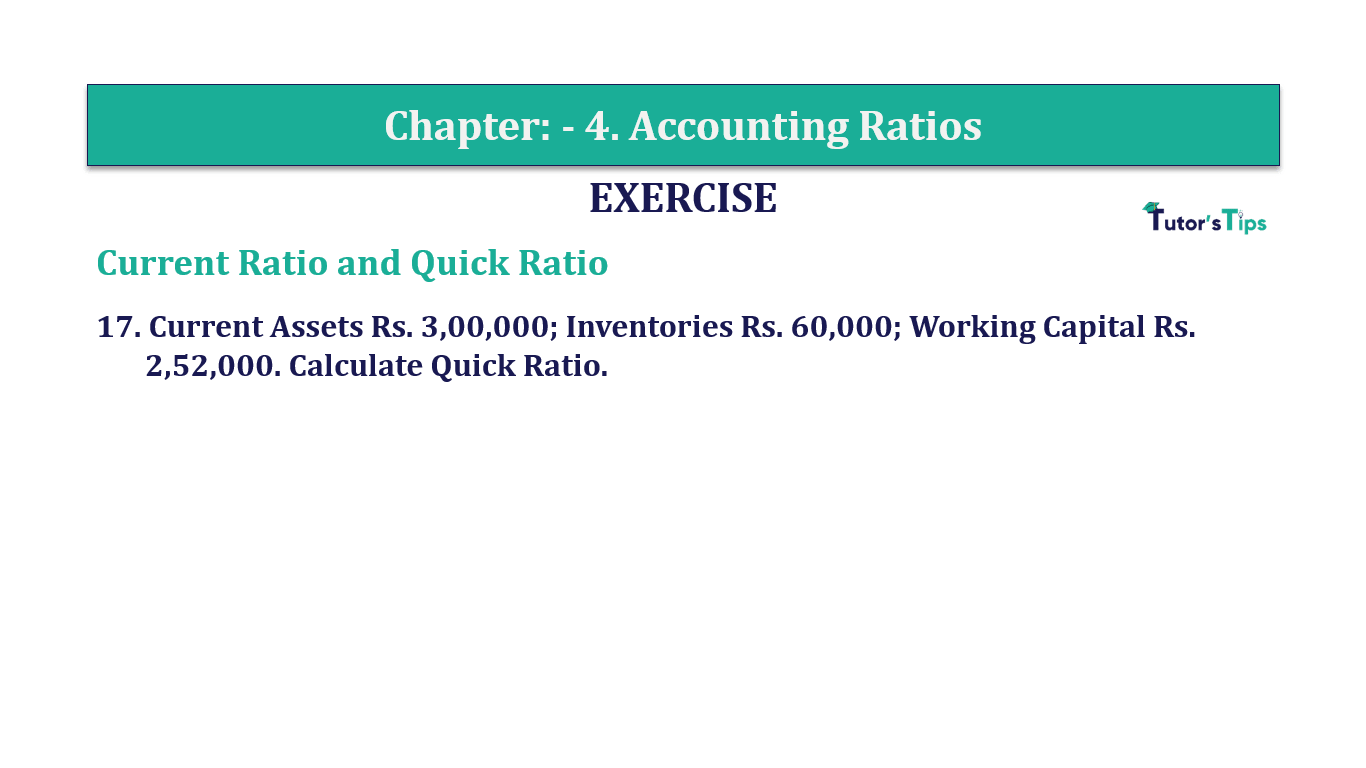# Question 17 Chapter 4 of +2-B – T.S. Grewal 12 ClassQuestion No. 17 - Chapter No.4 - T.S. Grewal +2 Book Part B

Question 17 Chapter 4 of +2-B

Current Ratio and Quick Ratio

17. Current Assets Rs. 3,00,000; Inventories Rs. 60,000; Working Capital Rs.
2,52,000. Calculate Quick Ratio.

### The solution of Question 17 Chapter 4 of +2-B: –

 Quick Assets = Rs.3,00,000 Inventory = Rs.60,000 Working Capital = Rs.2,52,000 Current Liabilities = Current Assets – Working Capital = Rs. 3,00,000 + Rs. 2,52,000 = Rs.48,000

 Quick Assets = Current Assets – Inventories = Rs.3,00,000 – Rs. 60,000 Quick Assets = Rs.2,40,000
 Current Ratio = Current Assets Current Liabilities
 = Rs.2,40,000 Rs.48,000

Quick Ratio or Liquid Ratio= 5 : 1

Balance Sheet: Meaning, Format & Examples

Comment if you have any question.

Also, Check out the solved question of previous Chapters: –